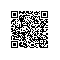# PHP SQL 注入攻击的技术实现以及预防办法

1. php 配置文件 php.ini 中的 magic_quotes_gpc 选项没有打开，被置为 off

2. 开发者没有对数据类型进行检查和转义

不过事实上，第二点最为重要。我认为, 对用户输入的数据类型进行检查，向 MYSQL 提交正确的数据类型，这应该是一个 web 程序员最最基本的素质。但现实中，常常有许多小白式的 Web 开发者忘了这点, 从而导致后门大开。

为什么说第二点最为重要？因为如果没有第二点的保证，magic_quotes_gpc 选项，不论为 on，还是为 off，都有可能引发 SQL 注入攻击。下面来看一下技术实现：

## 一. magic_quotes_gpc = Off 时的注入攻击

magic_quotes_gpc = Off 是 php 中一种非常不安全的选项。新版本的 php 已经将默认的值改为了 On。但仍有相当多的服务器的选项为 off。毕竟，再古董的服务器也是有人用的。

当magic_quotes_gpc = On　时，它会将提交的变量中所有的 '(单引号)、"(双号号)、\(反斜线)、空白字符，都为在前面自动加上 \。下面是 php 的官方说明：

magic_quotes_gpc boolean

Sets the magic_quotes state for GPC (Get/Post/Cookie) operations. When magic_quotes are on, all ' (single-quote), " (double quote), (backslash) and NUL's are escaped with a backslash automatically

如果没有转义，即 off 情况下，就会让攻击者有机可乘。以下列测试脚本为例：

http://www.xiaohui.com/weekly/20070314.htm

<?
if ( isset($_POST["f_login"] ) ) { // 连接数据库... // ...代码略... // 检查用户是否存在$t_strUname = $_POST["f_uname"];$t_strPwd = $_POST["f_pwd"];$t_strSQL = "SELECT * FROM tbl_users WHERE username='$t_strUname' AND password = '$t_strPwd' LIMIT 0,1";
if ( $t_hRes = mysql_query($t_strSQL) )
{
// 成功查询之后的处理. 略...
}
}
?>
<body>
<form method=post action="">
</form>
</body>

在这个脚本中，当用户输入正常的用户名和密码，假设值分别为 zhang3、abc123，则提交的 SQL 语句如下：

SELECT * FROM tbl_users
WHERE username='zhang3' AND password = 'abc123' LIMIT 0,1 

SELECT * FROM tbl_users
WHERE username='zhang3' OR 1=1 #' AND password = 'abc123' LIMIT 0,1

由于 # 是 mysql中的注释符, #之后的语句不被执行，实现上这行语句就成了：

SELECT * FROM tbl_users
WHERE username='zhang3' OR 1=1

这样攻击者就可以绕过认证了。如果攻击者知道数据库结构，那么它构建一个 UNION SELECT，那就更危险了：

假设在 username 中输入：zhang3 ' OR 1 =1 UNION select cola, colb,cold FROM tbl_b #

则提交的 SQL 语句变成：

SELECT * FROM tbl_users
OR 1 =1 UNION select cola, colb,cold FROM tbl_b #' AND password = 'abc123' LIMIT 0,1

这样就相当危险了。如果agic_quotes_gpc选项为 on，引号被转义，则上面攻击者构建的攻击语句就会变成这样，从而无法达到其目的：

SELECT * FROM tbl_users
LIMIT 0,1
SELECT * FROM tbl_users
WHERE username='zhang3 \' OR 1 =1 UNION select cola, colb,cold FROM tbl_b #'
AND password = 'abc123' LIMIT 0,1

## 二. magic_quotes_gpc = On 时的注入攻击

当 magic_quotes_gpc = On 时，攻击者无法对字符型的字段进行 SQL 注入。这并不代表这就安全了。这时，可以通过数值型的字段进行SQL注入。

在最新版的 MYSQL 5.x 中，已经严格了数据类型的输入，已默认关闭自动类型转换。数值型的字段，不能是引号标记的字符型。也就是说，假设 uid 是数值型的，在以前的 mysql 版本中，这样的语句是合法的：

INSERT INTO tbl_user SET uid="1";
SELECT * FROM tbl_user WHERE uid="1";

在最新的 MYSQL 5.x 中，上面的语句不是合法的，必须写成这样：

INSERT INTO tbl_user SET uid=1;
SELECT * FROM tbl_user WHERE uid=1;

这样我认为是正确的。因为作为开发者，向数据库提交正确的符合规则的数据类型，这是最基本的要求。

那么攻击者在 magic_quotes_gpc = On 时，他们怎么攻击呢？很简单，就是对数值型的字段进行 SQL 注入。以下列的 php 脚本为例：

<?
if ( isset($_POST["f_login"] ) ) { // 连接数据库... // ...代码略... // 检查用户是否存在$t_strUid = $_POST["f_uid"];$t_strPwd = $_POST["f_pwd"];$t_strSQL = "SELECT * FROM tbl_users WHERE uid=$t_strUid AND password = '$t_strPwd' LIMIT 0,1";
if ( $t_hRes = mysql_query($t_strSQL) )
{
// 成功查询之后的处理. 略...
}
}
?>
<body>
<form method=post action="">
User ID: <input type="text" name="f_uid" size=30><br>
</form>
</body>

上面这段脚本要求用户输入 userid 和 password 登入。一个正常的语句，用户输入 1001和abc123，提交的 sql 语句如下：

SELECT * FROM tbl_users WHERE userid=1001 AND password = 'abc123' LIMIT 0,1

如果攻击者在 userid 处，输入：1001 OR 1 =1 #，则注入的sql语句如下：

SELECT * FROM tbl_users WHERE userid=1001 OR 1 =1 # AND password = 'abc123' LIMIT 0,1

攻击者达到了目的。

## 三. 如何防止 PHP SQL 注入攻击

如何防止 php sql 注入攻击？我认为最重要的一点，就是要对数据类型进行检查和转义。总结的几点规则如下：

1. php.ini 中的 display_errors 选项，应该设为　display_errors = off。这样 php 脚本出错之后，不会在 web 页面输出错误，以免让攻击者分析出有作的信息。
2. 调用 mysql_query 等 mysql 函数时，前面应该加上 @，即 @mysql_query(...)，这样 mysql 错误不会被输出。同理以免让攻击者分析出有用的信息。另外，有些程序员在做开发时，当 mysql_query出错时，习惯输出错误以及 sql 语句，例如：
$t_strSQL = "SELECT a from b...."; if ( mysql_query($t_strSQL) )
{
// 正确的处理
}
else
{
echo "错误! SQL 语句：$t_strSQL \r\n错误信息".mysql_query(); exit; } 这种做法是相当危险和愚蠢的。如果一定要这么做，最好在网站的配置文件中，设一个全局变量或定义一个宏，设一下 debug 标志： 全局配置文件中： define("DEBUG_MODE",0); // 1: DEBUG MODE; 0: RELEASE MODE //调用脚本中：$t_strSQL = "SELECT a from b....";
if ( mysql_query($t_strSQL) ) { // 正确的处理 } else { if (DEBUG_MODE) echo "错误! SQL 语句：$t_strSQL \r\n错误信息".mysql_query();
exit;
}
3. 对提交的 sql 语句，进行转义和类型检查

## 四. 我写的一个安全参数获取函数

为了防止用户的错误数据和 php + mysql 注入 ，我写了一个函数 PAPI_GetSafeParam()，用来获取安全的参数值：

define("XH_PARAM_INT",0);
define("XH_PARAM_TXT",1);
function PAPI_GetSafeParam($pi_strName,$pi_Def = "", $pi_iType = XH_PARAM_TXT) { if ( isset($_GET[$pi_strName]) )$t_Val = trim($_GET[$pi_strName]);
else if ( isset($_POST[$pi_strName]))
$t_Val = trim($_POST[$pi_strName]); else return$pi_Def;
// INT
if ( XH_PARAM_INT == $pi_iType) { if (is_numeric($t_Val))
return $t_Val; else return$pi_Def;
}
// String
$t_Val = str_replace("&", "&amp;",$t_Val);
$t_Val = str_replace("<", "&lt;",$t_Val);
$t_Val = str_replace(">", "&gt;",$t_Val);
if ( get_magic_quotes_gpc() )
{
$t_Val = str_replace("\\\"", "&quot;",$t_Val);
$t_Val = str_replace("\\''", "'",$t_Val);
}
else
{
$t_Val = str_replace("\"", "&quot;",$t_Val);
$t_Val = str_replace("'", "'",$t_Val);
}
return $t_Val; } 在这个函数中，有三个参数： $pi_strName: 变量名
$pi_Def: 默认值$pi_iType: 数据类型。取值为 XH_PARAM_INT, XH_PARAM_TXT, 分别表示数值型和文本型。

如果请求是数值型，那么调用 is_numeric() 判断是否为数值。如果不是，则返回程序指定的默认值。

简单起见，对于文本串，我将用户输入的所有危险字符（包括HTML代码），全部转义。由于 php 函数 addslashes()存在漏洞，我用 str_replace()直接替换。get_magic_quotes_gpc() 函数是 php 的函数，用来判断 magic_quotes_gpc 选项是否打开。

刚才第二节的示例，代码可以这样调用：

<?
if ( isset($_POST["f_login"] ) ) { // 连接数据库... // ...代码略... // 检查用户是否存在$t_strUid = PAPI_GetSafeParam("f_uid", 0, XH_PARAM_INT);
$t_strPwd = PAPI_GetSafeParam("f_pwd", "", XH_PARAM_TXT);$t_strSQL = "SELECT * FROM tbl_users WHERE uid=$t_strUid AND password = '$t_strPwd' LIMIT 0,1";
if ( $t_hRes = mysql_query($t_strSQL) )
{
// 成功查询之后的处理. 略...
}
}
?>

这样的话，就已经相当安全了。PAPI_GetSafeParam的代码有点长，但牺牲这点效率，对保证安全，是值得的。希望大家多批评指正。：）使用钉钉扫一扫加入圈子
+ 订阅